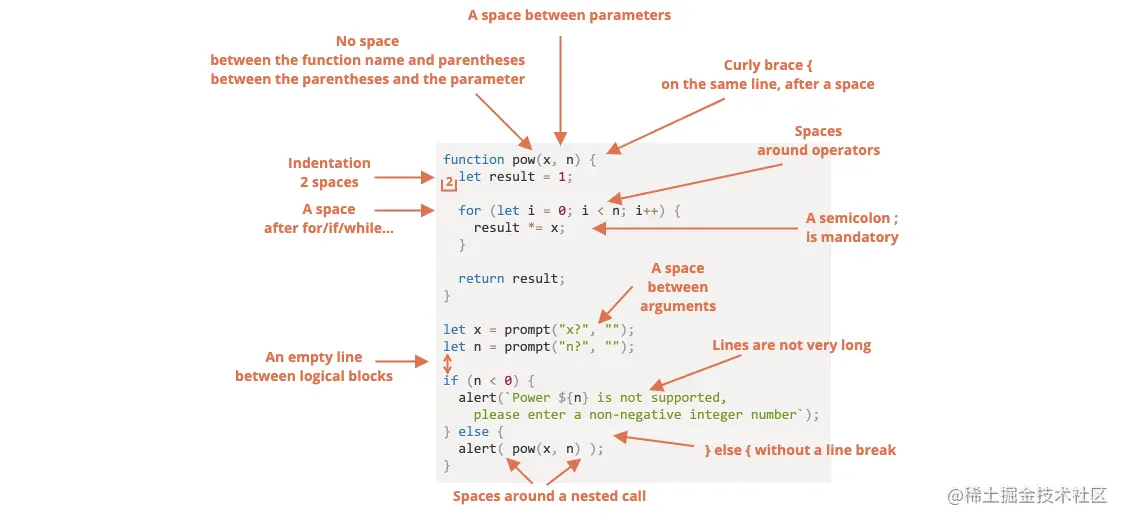# JS practical review notes (6)

2021-08-26 00:37:10 XiLan

## 1、 Browser debugging

F12

As we can see , There are three main ways to pause a script ：

1. A breakpoint . （ Call directly on the console ）
2. The `debugger` Statement .（ Write... In the code debugger ）
3. error （ If the development tool is open and the button    yes “ open ”）.

## 2、 Write code Partial specification

1、 Of course, you can configure it directly first vscode prettier When saving Just format the code1. Beginners sometimes do this .this is wrong , You don't need curly braces ：

``````if (n < 0)  { alert(`Power \${n} is not supported`); }
Copy code ``````
2. Split into separate lines , Without curly braces . Never do this , Error prone when adding new rows ：

``````if (n < 0)
Copy code ``````
3. A line without braces - Acceptable , If it's short ：

``````if (n < 0) alert(`do somethings`);
Copy code ``````

2、` A long part Show it in multiple lines ` As shown below ：

``````test(data1,
data2,
data3,
data4,
another
) {
// ...
}
Copy code ``````

3、 Boundary Write first ` The boundary conditions `

• this is wrong
``````for (let i = 0; i < 6; i++) {
if (code) {
... //
}
}
Copy code ``````
• this is right
``````for (let i = 0; i < 6; i++) {
if (!code) continue;
...  //
}
Copy code ``````

github.com/airbnb/java…

4、 Code checking Use `eslint ` Need configuration .eslintrc

• Write simple Easy to read accord with Standard code It's just Pursuit

## 3、 Write notes

1、 Good code should Basically no comments are needed Follow the method You might know what you're doing

2、` The functions in a function Disassemble into multiple function `

Sometimes it's useful to replace code snippets with functions , As shown below ：( Not split )

``````function test(n) {
nextP:
for (let i = 2; i < n; i++) {
for (let j = 2; j < n; j++) {
if (i % j == 0) continue nextP;
}

}
}
Copy code ``````
• this is right

A better variant , With decomposition function `isPrime`

``````function test(n) {

for (let i = 2; i < n; i++) {
if (!nextP(i)) continue;

}
}

function nextP(n) {
for (let i = 2; i < n; i++) {
if (n % i == 0) return false;
}

return true;
}
Copy code ``````

3、 Good annotation structure

• Record function parameters and usage

There is a special grammar JSDoc To record functions ： usage 、 Parameters 、 Return value .

for example ：

``````/**
* Returns x raised to the n-th power.
*
* @param {number} x The number to raise.
* @param {number} n The power, must be a natural number.
* @return {number} x raised to the n-th power.
*/
function pow(x, n) {
...
}
Copy code ``````

## 4、 Other specifications

1、 name Don't abbreviate

• `list`→ `lst`.
• `userAgent`→ `ua`.
• `browser`→ `brsr`.

2、 When selecting a name , Try to use the most abstract words . image `obj`,`data`,`value`,`item`,`elem` etc.

3、 Name the function As far as possible Named after the action of this function ` It starts with lowercase `

4、 Don't Use at will Underline naming It can lead to Confused I wonder if this is Special usage lodash With the exception of （ There is a library _ ）

5、 The code needs to be tested , There is an automated test library , Let's talk about it later